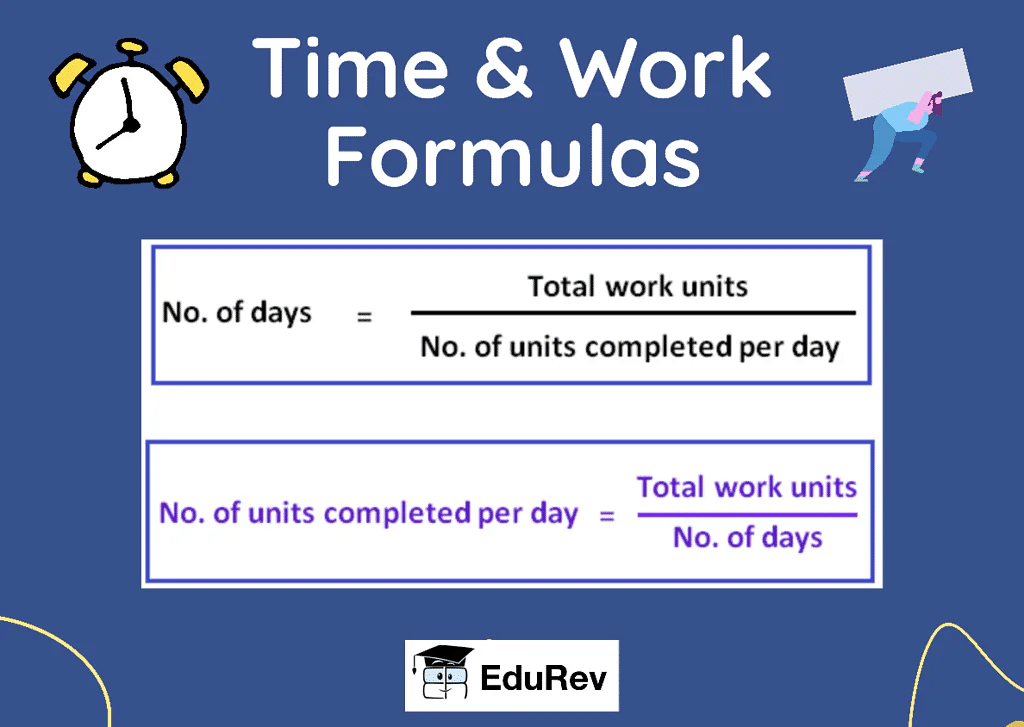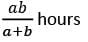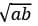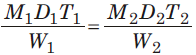UPSC  >  Important Formulas & Tips: Time & Work

# Important Formulas & Tips: Time & Work Notes | Study CSAT Preparation for UPSC CSE - UPSC

## Document Description: Important Formulas & Tips: Time & Work for UPSC 2022 is part of CSAT Preparation for UPSC CSE preparation. The notes and questions for Important Formulas & Tips: Time & Work have been prepared according to the UPSC exam syllabus. Information about Important Formulas & Tips: Time & Work covers topics like Formulas Used:&nbsp;, Some Tips to Remember:&nbsp;, Solved Questions: Time and Work, Time and Work and Important Formulas & Tips: Time & Work Example, for UPSC 2022 Exam. Find important definitions, questions, notes, meanings, examples, exercises and tests below for Important Formulas & Tips: Time & Work.

Introduction of Important Formulas & Tips: Time & Work in English is available as part of our CSAT Preparation for UPSC CSE for UPSC & Important Formulas & Tips: Time & Work in Hindi for CSAT Preparation for UPSC CSE course. Download more important topics related with notes, lectures and mock test series for UPSC Exam by signing up for free. UPSC: Important Formulas & Tips: Time & Work Notes | Study CSAT Preparation for UPSC CSE - UPSC
 Table of contents Formulas Used: Some Tips to Remember: Solved Questions: Time and Work Time and Work
 1 Crore+ students have signed up on EduRev. Have you?

Formulas Used:

Before you solve the questions, it is important that you are well aware of the basic concept, standard formulas, and types of questions for the same.1. Work Done = Time Taken × Rate of Work
2. Rate of Work = 1 / Time Taken
3. Time Taken = 1 / Rate of Work
4. If A can do a piece of work in n days, work done by A in 1 day = 1/n
If A does 1/n work in a day, A can finish the work in n days.
5. Total Work Done = Number of Days × Efficiency
6. Efficiency and Time are inversely proportional to each other
7. A does a particular job in ‘a’ hours and B does the same job in ‘b’ hours, together they will take:8. A does a particular job in ‘a’ hours more than A and B combined whereas B does the same job in ‘b’ hours more than A and B combined, then together they will takehours to finish the job.
9. Total Work Done = Number of Days × Efficiency
10. x:y is the ratio of the number of men who are required to complete a piece of work, then the ratio of the time taken by them to complete the work will be y:x
11. If M1 men can do W1 work in D1 days with T1 working hours/day and M2 men can do W2 work in D2 days with T2 working hours/day, then:Question for Important Formulas & Tips: Time & Work
Try yourself:A can do a work in 15 days and B in 20 days. If they work on it together for 4 days, then the fraction of the work that is left is :

Some Tips to Remember:

• A does a particular job in ‘a’ hours, B does the same job in ‘b’ hours and C does the same job in ‘c’ hours, then together they will take (abc/ab+bc+ca) hours.
• If A does a particular job in ‘a’ hours and A&B together do the job in ‘t’ hours, the B alone will take (at/a - t) hours.
• If A does a particular job in ‘a’ hours, B does the same job in ‘b’ hours and ABC together do the job in ‘t’ hours, then
⇒ C alone can do it in (abt/ab - at - bt) hours
⇒ A and C together can do it in (bt/b - t) hours
⇒ B and C together can do it in (at/a - t) hours
• If the objective is to fill the tank, then the Inlet pipes do positive work whereas the Outlet pipes do negative work.
If the objective is to empty the tank, then the Outlet pipes do positive work whereas the Inlet Pipes do negative work.

Question for Important Formulas & Tips: Time & Work
Try yourself:A works twice as fast as B. If B can complete a work in 12 days independently, the number of days in which A and B can together finish the work in :

Solved Questions: Time and Work

• All the hard work can go in vain if the candidate does not solve questions based on time and work on a regular basis and try using all the different formulas to crack the solution for each question.
• Below are a few time and work questions to give an idea as to what type of questions are asked in the competitive exam and what format and pattern are used for the same.

Q 1. A builder appoints three construction workers Akash, Sunil and Rakesh to one of his sites. They take 20, 30 and 60 days respectively to do a piece of work. How many days will it take Akash to complete the entire work if he is assisted by Sunil and Rakesh every third day?

1. 10 days
2. 15 days
3. 25 days
4. 30 days
5. 45 days

Solution:

Answer: (2) 15 days

Total work done by Akash, Sunil and Rakesh in 1 day = {(1/20) + (1/30) + (1/60)} = 1/10

Work done along by Akash in 2 days = (1/20) × 2 = 1/10

Work Done in 3 days (1 day of all three together + 2 days of Akash’s work) = (1/10) + (1/10) = 1/5

So, work done in 3 days = 1/5

Time taken to complete the work = 5×3 = 15 days

Q 2. To complete a piece of work, Samir takes 6 days and Tanvir takes 8 days alone respectively. Samir and Tanvir took Rs.2400 to do this work. When Amir joined them, the work was done in 3 days. What amount was paid to Amir?

1. Rs. 300
2. Rs. 400
3. Rs. 800
4. Rs. 500
5. Rs. 100

Solution:

Total work done by Samir and Tanvir = {(1/6) + (1/8)} = 7/24

Work done by Amir in 1 day = (1/3) – (7/24) = 1/24

Amount distributed between each of them =  (1/6) : (1/8) : (1/24) = 4:3:1

Amount paid to Amir = (1/24) × 3 × 2400 = Rs.300

Q 3. Dev completed the school project in 20 days. How many days will Arun take to complete the same work if he is 25% more efficient than Dev?

1. 10 days
2. 12 days
3. 16 days
4. 15 days
5. 5 days

Solution:

Answer: (3) 16 days

Let the days taken by Arun to complete the work be x

The ratio of time taken by Arun and Dev = 125:100 = 5:4

5:4 :: 20:x

⇒ x = {(4×20) / 5}

⇒ x = 16

Q 4. Time taken by A to finish a piece of work is twice the time taken B and thrice the time taken by C. If all three of them work together, it takes them 2 days to complete the entire work. How much work was done by B alone?

1. 2 days
2. 6 days
3. 3 days
4. 5 days
5. Cannot be determined

Solution:

Answer: (2) 6 days

Time taken by A  = x days

Time taken by B = x/2 days

Time Taken by C = x/3 days

⇒ {(1/x) + (2/x) + (3/x) = 1/2

⇒ 6/x = 1/2

⇒ x = 12

Time taken by B = x/2 = 12/2 = 6 days

Q 5. Sonal and Preeti started working on a project and they can complete the project in 30 days. Sonal worked for 16 days and Preeti completed the remaining work in 44 days. How many days would Preeti have taken to complete the entire project all by herself?

1. 20 days
2. 25 days
3. 55 days
4. 46 days
5. 60 days

Solution:

Answer: (5) 60 days

Let the work done by Sonal in 1 day be x

Let the work done by Preeti in 1 day be y

Then, x+y = 1/30 ——— (1)

⇒ 16x + 44y = 1  ——— (2)

Solving equation (1) and (2),

x = 1/60

y = 1/60

Thus, Preeti can complete the entire work in 60 days

The document Important Formulas & Tips: Time & Work Notes | Study CSAT Preparation for UPSC CSE - UPSC is a part of the UPSC Course CSAT Preparation for UPSC CSE.
All you need of UPSC at this link: UPSC

## CSAT Preparation for UPSC CSE

72 videos|64 docs|92 tests
 Use Code STAYHOME200 and get INR 200 additional OFF

## CSAT Preparation for UPSC CSE

72 videos|64 docs|92 tests

### How to Prepare for UPSC

Read our guide to prepare for UPSC which is created by Toppers & the best Teachers

Track your progress, build streaks, highlight & save important lessons and more!

,

,

,

,

,

,

,

,

,

,

,

,

,

,

,

,

,

,

,

,

,

;